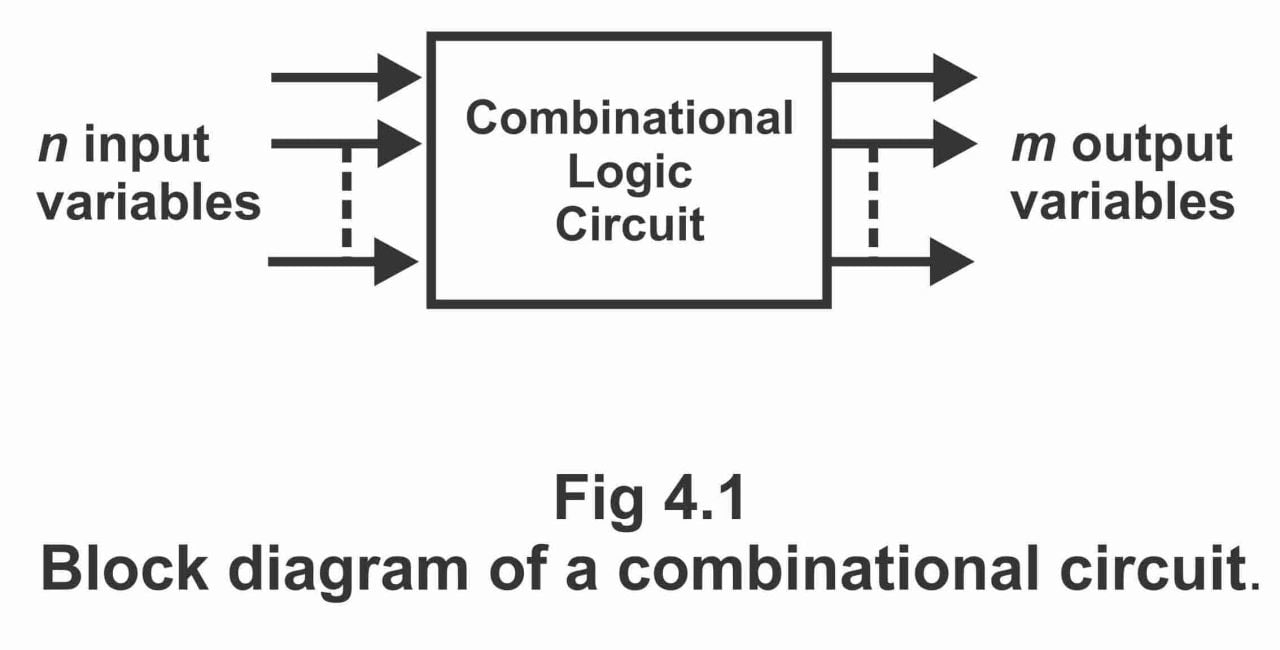Digital Electronics

# Combinational logic & Data Processing circuits

## Combinational logic & Data Processing circuits

A combination of inter-connected logic gates, which can perform a specific Boolean function without having the capability of memory or storage, is known as a combinational logic circuit. In other words, a combinational logic circuit consists of such logic gates, the outputs of which can directly be determined at any time through the present inputs’ combination without keeping in mind the previous inputs. As a combinational circuit performs the function of processing data or specific information through a set of Boolean functions in a logical state, thus circuits designed by means of combinational logic circuits, are also known as data processing circuits. In other words, as binary data process during various operations of combinational circuits, (a series of different operations in order to get certain distinct results, is called the process) therefore, such combinational circuits are also known as data processing circuits.

Hence, combinational logic circuits are such circuits, the output of which is solely depends on values of the present combinations of variables, and when input combinations change, output also changes. The output of combinational logic circuit depends on the circuit’s input, the types of gates being used, and the various methods being adopted for connecting these gates. It has to be remembered that these circuits are generally available in the form of MSI integrated circuits. As combinational logic circuits are designed through a combination of logical gates (as the name implies) therefore, they are commonly used in solving logical problems e.g. multiplexing, encoding, and decoding, etc.

A combinational circuit consists of input variables, logic gates, and output variables. Logic gates receive signals from inputs and create signals on outputs. As a result of this process, binary information changes from a given input data towards required output data. In general, both input and output data are represented through binary signals (or two possible states or values i.e. logic 1 and logic 0). The block diagram of a combinational circuit has been shown in figure 4.1, according to which “n” input binary variables are received by the circuit through some external source, whereas output variables “m” received from this circuit go towards some other external circuit or source. These external sources during various applications are normally storage registers, which remain nearby a combinational circuit or exist on some far–off the device. It should be remembered that every input variable of every combinational circuit contains one or two wires. When only one wire is available, it shows that the variable is in the normal state or it is in complement form. As one of the variables in a Boolean expression is normal or complement, therefore it is necessary to provide an inverter on the input wire. On the contrary, in case the input variable is reflected via two wires, the circuit’s input will have both normal as well as complement states. Thus, under such a situation, it is not incumbent to include an inverter along with inputs.

Figure 4.1 – block diagram of a combinational circuitPrevious Topic: Basic comparator operations with circuit diagram examples

For electronics and programming-related projects visit my YouTube channel.